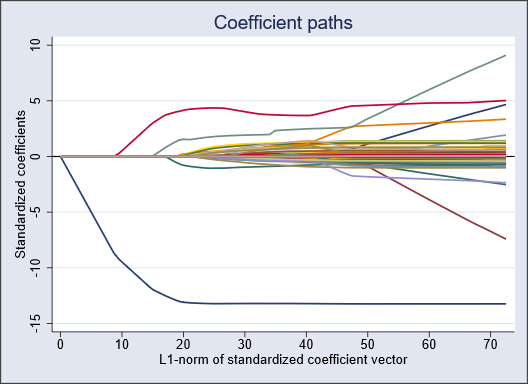Lasso for prediction and model selection

Lasso

Elastic net

Square-root lasso

Continuous, binary, and count outcomes

Lasso for inference

Effect estimates for covariates of interest

Coefficients, SEs, tests, confidence intervals

Lasso selects control variables

Robust to model-selection mistakes by lasso

Double selection

Partialing out

Cross-fit partialing out

Double machine learning (DML1 and DML2)

Linear, logit, and Poisson regression

Endogenous covariates in linear models

Data preparation

Divide data into random samples

Manage large lists of variables

Create names for referring to

All continuous variables

All categorical variables

Your own variable groupings

Selection methods

Cross-validation

Plugin iterative formula

Evaluate selected model

Graphs

Cross-validation function plot

Coefficient paths

Goodness of fit

Mean squared error of prediction

In-sample and out-of-sample R-squareds

Deviance

In-sample and out-of-sample deviance ratios

BIC

Relative L1-norm of coefficients

Relative L2-norm squared of coefficients

Estimated coefficients

Penalized

Postselection